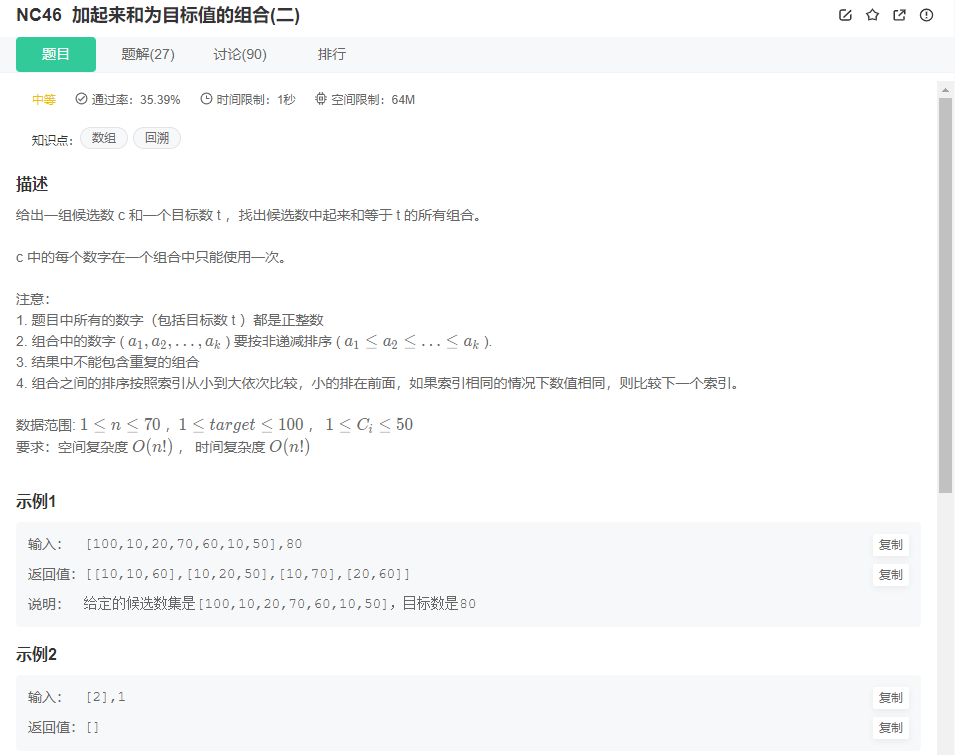NC46 加起来和为目标值的组合(二)

NC46 加起来和为目标值的组合(二)//这里并不是题解，而是求出数组数字所有的组合  例如 [1，2，3] -> [,[1,2],[1,2,3],,[2,3],]
//求 数组中任意组合的和==target   就需要先列出所有的组合。先看懂这段代码。
public class NC46 {
//存储结果集
static ArrayList<ArrayList<Integer>> result = new ArrayList<>();
//存储选中的序列，符合条件 则将改序列加入 到result
static ArrayList<Integer> list = new ArrayList<>();
public static void dfs(int[] nums, int start) {
for (int i = start; i < nums.length; i++) {
dfs(nums,  i + 1);
list.remove(list.size() - 1);
}
}
public static ArrayList<ArrayList<Integer>> combinationSum2(int[] nums, int t) {
Arrays.sort(nums);
dfs(nums,  0);
return result;
}
public static void main(String[] args) {
int[] a = {1, 2,3};
combinationSum2(a, 2);
}

}
//题解
public class NC46 {
//存储结果集
static ArrayList<ArrayList<Integer>> result = new ArrayList<>();
//存储选中的序列，符合条件 则将改序列加入 到result
static ArrayList<Integer> list = new ArrayList<>();

public static void dfs(int[] nums, int target, int start) {
if (target == 0) {
// 列表元素之和 == target  ，将列表加入 result
return;
}

if (start >= nums.length)
return;

for (int i = start; i < nums.length; i++) {
//nums是有序数组，当nums[i]
//如果把这个条件注释掉  对于 [1,1,1,2],3    输出的结果是 [[1,1,1],[1,2],[1,2],[1,2]]
if (i > start && nums[i] == nums[i - 1])
continue;
//剪枝。 跳过该元素。
if (nums[i] > target)
continue;
//递归，接下来利用剩余的数组 求 目标值  =   target - nums[i]
dfs(nums, target - nums[i], i + 1);
list.remove(list.size() - 1);
}

}

public static ArrayList<ArrayList<Integer>> combinationSum2(int[] nums, int t) {
//数组是升序的 后续结论都是基于有序数组。
Arrays.sort(nums);
dfs(nums,t,0);
return result;
}

public static void main(String[] args) {
int[] a = {1,1,1,2};
combinationSum2(a, 2);
}

}
if (i > start && nums[i] == nums[i - 1])    【后续这段判断用‘条件1’代指】
continue;

这段代码需要仔细斟酌一下。 到底是怎么做到去重的。(用这个判断条件，需要数组是有序的，数组一开始已经排过序了。)
以测试用例 [1,1,1,2],2 为例 输出应该是[[1,1],]  如果没有这段代码则输出 [[1,1],[1,1],[1,1],]
也就是将每个1都当作了不同的元素处理

if (nums[i] > target)    【剪枝， 用‘条件2’指代】
continue;

首先调用  combinationSum2(a, 2);

-> target=2 start=0 i=0      list=
-> 继续调用dfs   target=1   start=1  i=1   条件1=false     list=[1,1]
->dfs target=0 start=2 i=2  target == 0 条件满足  将[1,1]加入result.  调用结束 return;
-> list.remove(list.size() - 1); 移除list中刚在本次dfs加入的元素    此时 list=
-> target =1 start=1 i=2 条件1=true continue
-> target =1 start=1 i=3  此时num=2 大于 target=1 ，条件2=true(即后续继续dfs()也凑不出target)  ，直接跳过这层循环。 该层递归结束 return
-> list.remove(list.size() - 1); 此时list=[]
-> target=2 start=0 i=1 条件1=true continue;
-> target=2 start=0 i=2 条件1=true continue;
-> target=2 start=0 i=3 条件1=false 条件2=false
-> target=0 start=4      此时target==0 list= 加入result 然后 return;
-> list.remove(list.size() - 1); 循环至此结束 首次调用的dfs return;

在首次进入dfs时， i=0 就是将nums 取出，    然后求后续任意  target-nums[i] 的组合
i=1  取出nums ，再求后续 target-nums的组合
这样一层层循环 ，每层循环递归 就能排列出所有组合。

那为什么会重复呢？
当nums 和 nums相等的时候 ，   nums+剩余组合   和  nums+ 剩余组合 是有重复的。
比如 [1,1,1,2]    会产生三个数组 [1,2] [1,2] [1,2]  这三个中我们只需要第一个nums=1存在的组合就够了 [1,2]
那包含nums=1 的组合会不会被去掉呢？即[1,1,1]这个组合怎么没有被去掉。
不会。  在i==0时  加入了nums 之后 ，再次进入dfs 此时nums即便与前面的数字相等也会被算进去。
总结：   nums+剩余数组的组合  和  nums+剩余数组的组合  在num==nums时  两个组合是有重复的。
其中前者是真包含后者的。 所以要加    if (i > start && nums[i] == nums[i - 1]) 这层判断。
07-081764
08-035558

11-16103
09-18430
08-257873
01-13320
11-17668
12-1923
07-10128
08-1962
04-1683
04-21150
01-14404lemon.V

¥2 ¥4 ¥6 ¥10 ¥20余额支付 (余额：-- )扫码支付获取中扫码支付点击重新获取扫码支付1.余额是钱包充值的虚拟货币，按照1:1的比例进行支付金额的抵扣。
2.余额无法直接购买下载，可以购买VIP、C币套餐、付费专栏及课程。余额充值